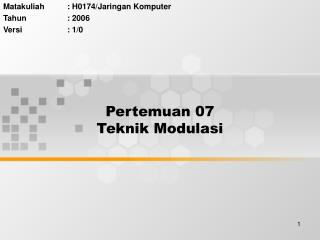DownloadDownload PresentationPertemuan 07 Teknik Modulasi

# Pertemuan 07 Teknik Modulasi

Télécharger la présentation## Pertemuan 07 Teknik Modulasi

- - - - - - - - - - - - - - - - - - - - - - - - - - - E N D - - - - - - - - - - - - - - - - - - - - - - - - - - -
##### Presentation Transcript

1. Matakuliah : H0174/Jaringan Komputer Tahun : 2006 Versi : 1/0 Pertemuan 07Teknik Modulasi

2. Learning Outcomes Pada akhir pertemuan ini, diharapkan mahasiswa akan mampu: • Menjelaskan teknik modulasi

3. Outline Materi • Teknik Modulasi • Modulasi analog • Modulasi digital

4. Encoding Techniques • Analog data, carrier analog signal • Digital data, carrier analog signal Analog Modulation • Analog data, carrier digital signal Digital Modulation • Digital data, carrier digital signal Encoding

5. Analog Data, Analog Signals • Why modulate analog signals? • Higher frequency can give more efficient transmission • Permits frequency division multiplexing • Types of modulation • Amplitude • Frequency • Phase

6. Analog Modulation

7. MODULASI TUJUAN: • MENGUBAH SINYAL DARI KOMPUTER YANG DIGITAL MENJADI SINYAL ANALOG YANG DIPERLUKAN OLEH SALURAN KOMUNIKASI • MEMPERPANJANG JARAK JANGKAU SINYAL INFORMASI • MODEM MERUPAKAN HARDWARE UNTUK KEDUA PROSES INI LST/FASKD/CINQ

8. Type Modulasi Digital /Analog

9. Modulation Techniques

10. Frequency Shift Keying • Most common form is binary FSK (BFSK) • Two binary values represented by two different frequencies (near carrier) • Less susceptible to error than ASK • Up to 1200bps on voice grade lines • High frequency radio • Even higher frequency on LANs using co-ax

11. Phase Shift Keying • Phase of carrier signal is shifted to represent data • Binary PSK • Two phases represent two binary digits • Differential PSK • Phase shifted relative to previous transmission rather than some reference signal

12. PSK Constellation

13. Quadrature PSK • More efficient use by each signal element representing more than one bit • e.g. shifts of /2 (90o) • Each element represents two bits • Can use 8 phase angles and have more than one amplitude • 9600bps modem use 12 angles , four of which have two amplitudes

14. 4-PSK Constellation

15. 4-QAM and 8-QAM

16. Quadrature Amplitude Modulation • QAM used on asymmetric digital subscriber line (ADSL) and some wireless • Combination of ASK and PSK • Logical extension of QPSK • Send two different signals simultaneously on same carrier frequency • Use two copies of carrier, one shifted 90° • Each carrier is ASK modulated • Two independent signals over same medium • Demodulate and combine for original binary output

17. BIT dan BAUD

18. Perbandingan Bit dan Baud rate

19. Matakuliah : H0174/Jaringan Komputer Tahun : 2006 Versi : 1/0 Pertemuan 08Teknik Encoding

20. Learning Outcomes Pada akhir pertemuan ini, diharapkan mahasiswa akan mampu : • Menjelaskan teknik encoding

21. Outline Materi • Polar • Bipolar • Unipolar

22. Digital to Digital Encoding

23. Terminologi • Unipolar • All signal elements have same sign • Polar • One logic state represented by positive voltage the other by negative voltage • Data rate • Rate of data transmission in bits per second • Duration or length of a bit • Time taken for transmitter to emit the bit

24. Terminologi • Modulation rate • Rate at which the signal level changes • Measured in baud = signal elements per second • Mark and Space • Binary 1 and Binary 0 respectively

25. Digital to Digital Encoding

26. Polar Encoding

27. Bipolar Encoding

28. Modulation Rate

29. Unipolar Encoding - NRZL

30. Nonreturn to Zero Inverted • Polar, Non Return To Zero Inverted • Non return to zero, inverted on ones • Constant voltage pulse for duration of bit • Data encoded as presence or absence of signal transition at beginning of bit time • Transition (low to high or high to low) denotes a binary 1 • No transition denotes binary 0 • An example of differential encoding

31. NRZ-L and NRZ-I

32. RZ Encoding

33. Biphase • Manchester • Transition in middle of each bit period • Transition serves as clock and data • Low to high represents one • High to low represents zero • Used by IEEE 802.3 • Differential Manchester • Mid bit transition is clocking only • Transition at start of a bit period represents zero • No transition at start of a bit period represents one • Used by IEEE 802.5

34. Manchester and Diff. Manchester

35. Multilevel Binary • Use more than two levels • Bipolar-AMI • zero represented by no line signal • one represented by positive or negative pulse • one pulses alternate in polarity • No loss of sync if a long string of ones (zeros still a problem) • No net dc component • Lower bandwidth • Easy error detection

36. Bipolar AMI Encoding

37. Perbandingan skema enkoding • Error detection • Can be built in to signal encoding • Signal interference and noise immunity • Some codes are better than others • Cost and complexity • Higher signal rate (& thus data rate) lead to higher costs • Some codes require signal rate greater than data rate